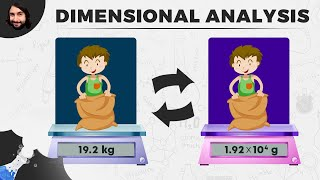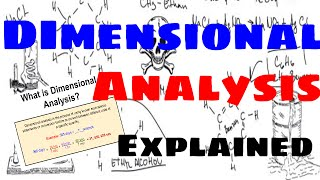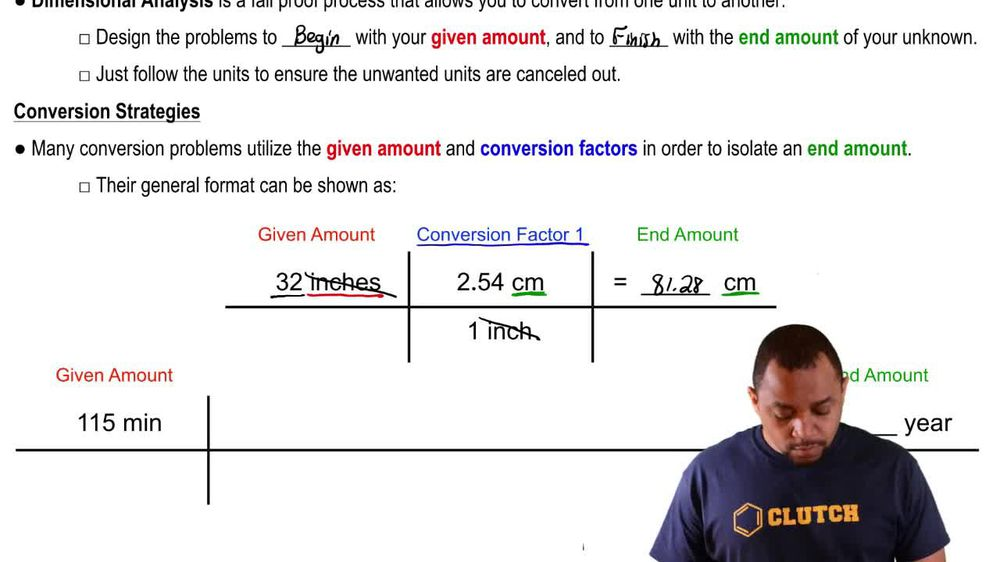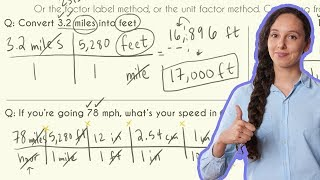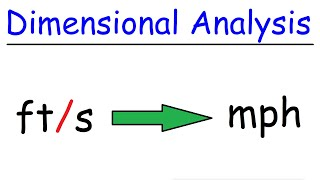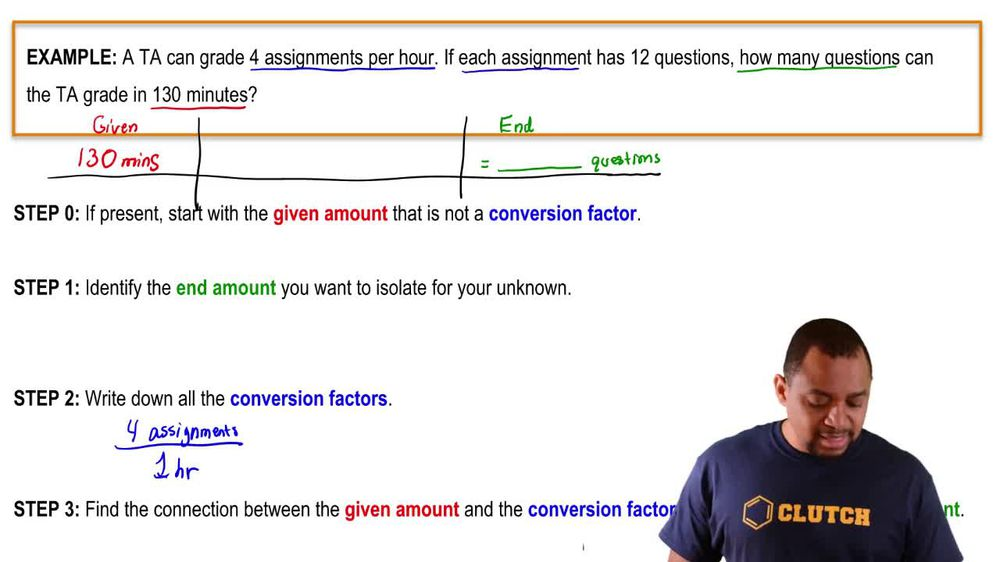Start typing, then use the up and down arrows to select an option from the list.
1. 1. Intro to General Chemistry2. Dimensional Analysis
Problem

# (a) Assuming the dimensions of the nucleus and atom shown in Figure 2.10, what fraction of the volume of the atom is taken up by the nucleus?

Relevant Solution3m
Play a video:
Hi everyone here we have a question telling us that atmospheric pressure and negative two degrees Celsius. There are 7.89 times 10 to the 21st atoms per liter of gaseous helium atoms, helium has a radius of 140 PICO meters determine the fraction of space occupied by the helium atoms. So our radius is going to equal PICO meters Times one deck a meter over 10 to the 11th. Pick a meters. So our PICO meters are going to cancel out and that's going to give us 1.40 times 10 to the negative ninth Decca meters. Our volume equals four pi Divided by three times r cubed. So our volume is going to equal four pi, divided by three times 1. times 10 to the negative ninth Decca meters cubed. It's our volume Equals 1. four 0403, two times To the native 26 Decca meters cubed times one liter over one decade meter cubed Equals 1.14940403, 2 times 10 to the - L. And now we need to calculate the fraction occupied by helium atoms. So that's going to be 1.149, times 10 to the negative 26 liters per adam Times 7.89 times 10 to the 21st Adams per leader, which equals 9.06, nine 7814 times 10 to the negative fifth or one divided by one 1000 .8. And if we look, we see that our leaders cancel out and our Adams cancel out. So this is unit Liss. And that is our final answer. Thank you for watching. Bye.